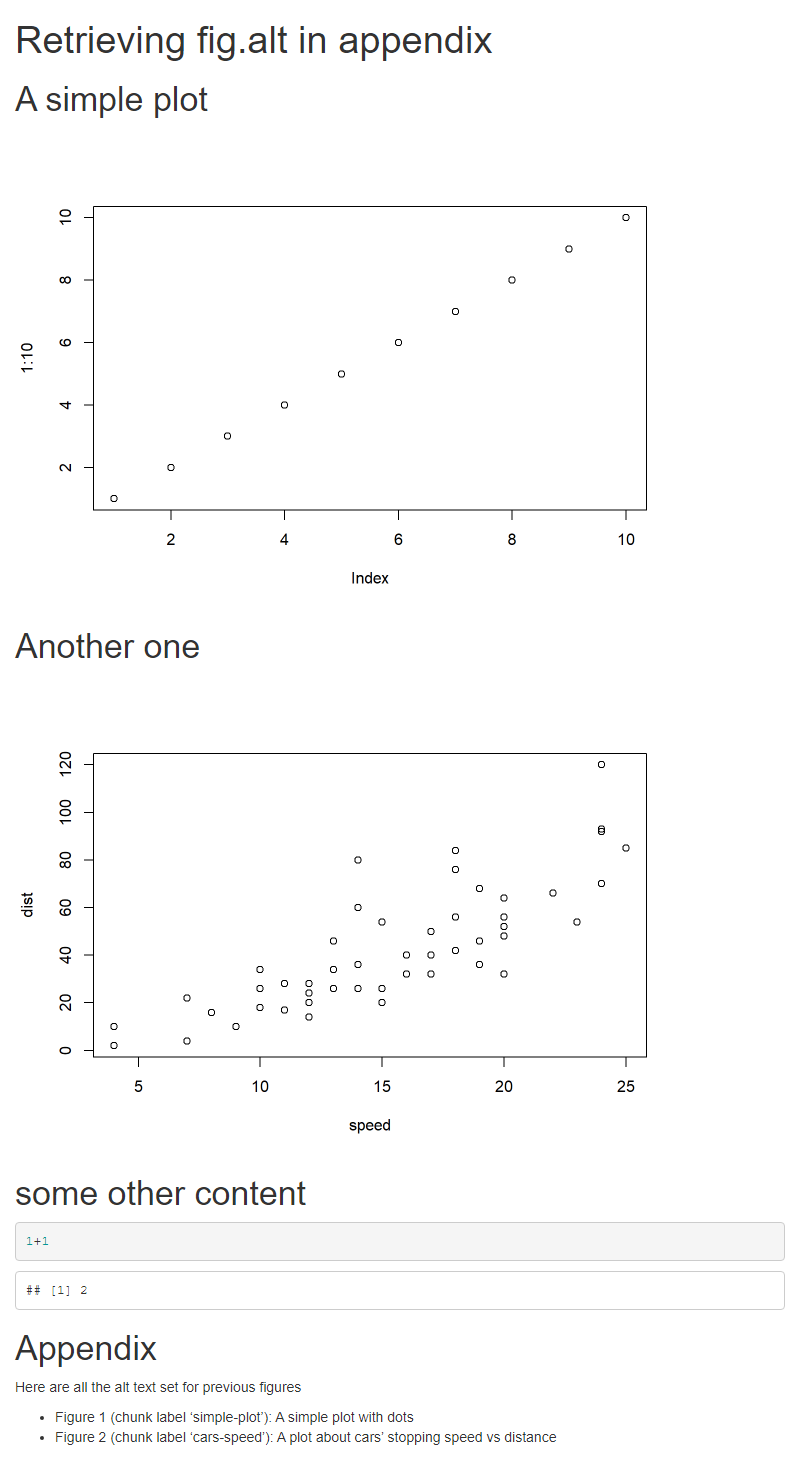Recently, I was pinged on Twitter about a ‘How to’ with rmarkdown and knitr

The idea is simple:

Can we retrieve all alternative texts set with `fig.alt` chunk option1 to display them in an appendix at the end of the document ?

There is not a straightforward way but using a few knitr tricks, this can be done. Let’s take this small example file:

``````---
title: Retrieving fig.alt in appendix
output: html_document
---``````
``````
# A simple plot

```{r simple-plot, fig.alt = "A simple plot with dots", echo = FALSE}
plot(1:10)
```

# Another one

```{r cars-speed, fig.alt = "A plot about cars' stopping speed vs distance", echo = FALSE}
plot(cars)
```
# some other content

```{r}
1+1
```

# Appendix

Here are all the alt text set for previous figures

``````

We would like to retrieve all the `fig.alt` set at the end of the document as a bullet point list. This can be done using advanced knitr features:

• `knitr::all_labels()` which allows to retrieve all chunk label in a document. You can find a usage example in the R Markdown Cookbook about how to Put together all code in the appendix
• `knitr::knit_code` which allows to retrieve code content from any chunk using its labels. The object return contains chunk options in attributes. This post by Emi Tanaka gives a nice introduction on what this can do. This is a really advanced feature to use with caution. See at leas `?knitr::knit_code` before using it!
• `result='asis'` and `knitr::knit_expand()` to generate Rmd source from variable.

Putting this together, we would do first

• Retrieve the chunks with a `fig.alt` labels
• Retrieve the options set on these chunks to get the `fig.alt` one
``````# get all labels from chunk with fig.alt chunk option defined
chunks_with_alt <- knitr::all_labels(nzchar(fig.alt))
# Use these labels to retrieve code content
chunks_content <- knitr::knit_code\$get(chunks_with_alt)
# retrieve specifically chunk options
chunks_opts <- lapply(chunks_content, function(x) attr(x, "chunk_opts"))``````

Then we would write our markdown content, for example using `knitr::knit_expand` as templating mechanism. We want something like this in markdown to obtain a list in HTML

``* Figure 1 (chunk label 'plot-1): An alt text``

This would be generated by looping on selected chunk option to create these markdown strings.

``````# Looping over the selected chunks
l <- lapply(seq_along(chunks_opts), function(opt_ind) {
# We use the index number but we need the option content
opt <- chunks_opts[[opt_ind]]
knitr::knit_expand(
# template string to fill in using index...
text = "* Figure {{opt_ind}} (chunk label '{{label}}'): {{alt_text}}",
# and the alt text set...
alt_text = opt\$fig.alt,
# and the label of the chunk
label = opt\$label)
})
# Using cat() to output the string content to be included as-is.
cat(unlist(l), sep = "\n")``````

The code above could be included in a single Rmd document like this, using the correct chunk option to hide the code and output the markdown strings as is.

``````---
title: Retrieving fig.alt in appendix
output: html_document
---``````
``````
# A simple plot

```{r simple-plot, fig.alt = "A simple plot with dots", echo = FALSE}
plot(1:10)
```

# Another one

```{r cars-speed, fig.alt = "A plot about cars' stopping speed vs distance", echo = FALSE}
plot(cars)
```

# some other content

```{r}
1+1
```

# Appendix

Here are all the alt text set for previous figures

```{r retrieve-chunk-opt, include= FALSE}
# get all labels from chunk with fig.alt chunk option defined
chunks_with_alt <- knitr::all_labels(nzchar(fig.alt))
# Use these labels to retrieve code content
chunks_content <- knitr::knit_code\$get(chunks_with_alt)
# retrieve specifically chunk options
chunks_opts <- lapply(chunks_content, function(x) attr(x, "chunk_opts"))
```

```{r write-appendix, results='asis', echo = FALSE}
# Looping over the selected chunks
l <- lapply(seq_along(chunks_opts), function(opt_ind) {
# We use the index number but we need the option content
opt <- chunks_opts[[opt_ind]]
knitr::knit_expand(
# template string to fill in using index...
text = "* Figure {{opt_ind}} (chunk label '{{label}}'): {{alt_text}}",
# and the alt text set...
alt_text = opt\$fig.alt,
# and the label of the chunk
label = opt\$label)
})
# Using cat() to output the string content to be included as-is.
cat(unlist(l), sep = "\n")
```
``````

After rendering, the resulting document would look like thisThis is a rather advanced solution using some of the less-known feature of knitr. I am sure there could also be another approach using the parsermd by `@rundel` which helps you parse a Rmd file, including chunk option. However, that would mean reading the Rmd file during rendering of the same Rmd file… but why not ! I let you try.

For more recipes about R Markdown, don’t forget to read the R Markdown Cookbook from which some of the tricks here are based !

1. If you are not familiar with this chunk option, this is a new one added in knitr 1.32 to set alternative text on any figures inside code chunk. More about it on the This blog post.↩︎